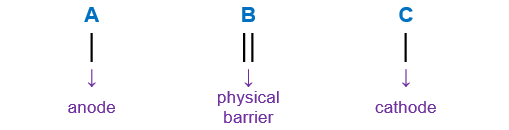# Problem: Write the overall balanced equation for the reaction:Mn(s)|Mn2+(aq) ∥ ClO2(g)|ClO−2(aq) | Pt(s)Then calculate  E°cell.

###### FREE Expert Solution

When writing a cell notation, we use the following format – “as easy as ABC*Pt is the electrode used in the cell and it is inert (doesn’t participate in the reaction) so it will not be part of the reaction equation

Anode Reaction:         Mn(s) → Mn2+(aq)

Cathode Reaction:     ClO2(g) → ClO2-(aq)

80% (306 ratings)###### Problem Details

Write the overall balanced equation for the reaction:

Mn(s)|Mn2+(aq) ∥ ClO2(g)|ClO−2(aq) | Pt(s)

Then calculate  E°cell.

Frequently Asked Questions

What scientific concept do you need to know in order to solve this problem?

Our tutors have indicated that to solve this problem you will need to apply the Cell Potential concept. If you need more Cell Potential practice, you can also practice Cell Potential practice problems.

What professor is this problem relevant for?

Based on our data, we think this problem is relevant for Professor Altomare's class at UCF.